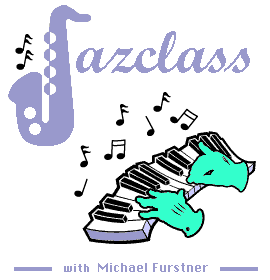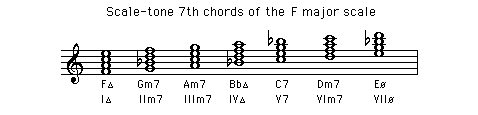Jazz Chords SCALE-TONE CHORDSof the major scale Description Scale-tone chordsC | Db | D | Eb | E | F | F# = Gb | G | Ab | A | Bb | B

(
Down - Top)

Scale-tone 7th Chords of the Major scale

Scale-tone seventh chords are chords formed by notes of a given scale. They contain therefore only notes that belong to that scale.

The scale-tone 7th chords of the major scale are :

Chord QualitySymbolC majorF majorG major
I major 7th

II minor 7th

III minor 7th

IV major 7th

V dominant 7th

VI minor 7th

VII half diminished

Imaj7

IIm7

IIIm7

IVmaj7

V7

VIm7

VIIø

C E G B

D F A C

E G B D

F A C E

G B D F

A C E G

B D F A

F A C E

G Bb D F

A C E G

Bb D F A

C E G Bb

D F A C

E G Bb D

G B D F#

A C E G

B D F# A

C E G B

D F# A C

E G B D

F# A C E

Audio 1The quality of these chords remain the same for chords of the major scale in all keys.

For example the second chord of the major scale in any key will always be a minor 7th chord, the fifth chord will always be a dominant 7th chord, and so on.
Below the scale-tone 7th chords for the F major scale.

Audio 2
(played one octave lower as written)(
Down - Up - Top)

The Scale-tone 7th Chords of the major scale in all 12 keys are listed below in Circle of Fifths order.

 C major scale-tone chords (Down - Up - Top) Cmaj7 = C - E - G - B Dm7 = D - F - A - C Em7 = E - G - B - D Fmaj7 = F - A - C - E G7 = G - B - D - F Am7 = A - C - E - G Bø = B - D - F - A G major scale-tone chords (Down - Up - Top) Gmaj7 = G - B - D - F# Am7 = A - C - E - G Bm7 = B - D - F# - A Cmaj7 = C - E - G - B D7 = D - F# - A - C Em7 = E - G - B - D F#ø = F# - A - C - E D major scale-tone chords (Down - Up - Top) Dmaj7 = D - F# - A - C# Em7 = E - G - B - D F#m7 = F# - A - C# - E Gmaj7 = G - B - D - F# A7 = A - C# - E - G Bm7 = B - D - F# - A C#ø = C# - E - G - B A major scale-tone chords (Down - Up - Top) Amaj7 = A - C# - E - G# Bm7 = B - D - F# - A C#m7 = C# - E - G# - B Dmaj7 = D - F# - A - C# E7 = E - G# - B - D F#m7 = F# - A - C# - E G#ø = G# - B - D - F# E major scale-tone chords (Down - Up - Top) Emaj7 = E - G# - B - D# F#m7 = F# - A - C# - E G#m7 = G# - B - D# - F# Amaj7 = A - C# - E - G# B7 = B - D# - F# - A C#m7 = C# - E - G# - B D#ø = D# - F# - A - C# B major scale-tone chords (Down - Up - Top) Bmaj7 = B - D# - F# - A# C#m7 = C# - E - G# - B D#m7 = D# - F# - A# - C# Emaj7 = E - G# - B - D# F#7 = F# - A# - C# - E G#m7 = G# - B - D# - F# A#ø = A# - C# - E - G# F# major scale-tone chords (Down - Up - Top) F#maj7 = F# - A# - C# - E#(=F) G#m7 = G# - B - D# - F# A#m7 = A# - C# - E#(=F) - G# Bmaj7 = B - D# - F# - A# C#7 = C# - E#(=F) - G# - B D#m7 = D# - F# - A# - C# E#ø = E#(=F) - G# - B - D# Gb major scale-tone chords (Down - Up - Top) Gbmaj7 = Gb - Bb - Db - F Abm7 = Ab - B - Eb - Gb Bbm7 = Bb - Db - F - Ab Cbmaj7 = Cb - Eb - Gb - Bb Db7 = Db - F - Ab - B Ebm7 = Eb - Gb - Bb - Db Fø = F - Ab - B - Eb Db major scale-tone chords (Down - Up - Top) Dbmaj7 = Db - F - Ab - C Ebm7 = Eb - Gb - Bb - Db Fm7 = F - Ab - C - Eb Gbmaj7 = Gb - Bb - Db - F Ab7 = Ab - C - Eb - Gb Bbm7 = Bb - Db - F - Ab Cø = C - Eb - Gb - Bb Ab major scale-tone chords (Down - Up - Top) Abmaj7 = Ab - C - Eb - G Bbm7 = Bb - Db - F - Ab Cm7 = C - Eb - G - Bb Dbmaj7 = Db - F - Ab - C Eb7 = Eb - G - Bb - Db Fm7 = F - Ab - C - Eb Gø = G - Bb - Db - F Eb major scale-tone chords (Down - Up - Top) Ebmaj7 = Eb - G - Bb - D Fm7 = F - Ab - C - Eb Gm7 = G - Bb - D - F Abmaj7 = Ab - C - Eb - G Bb7 = Bb - D - F - Ab Cm7 = C - Eb - G - Bb Dø = D - F - Ab - C Bb major scale-tone chords (Down - Up - Top) Bbmaj7 = Bb - D - F - A Cm7 = C - Eb - G - Bb Dm7 = D - F - A - C Ebmaj7 = Eb - G - Bb - D F7 = F - A - C - Eb Gm7 = G - Bb - D - F Aø = A - C - Eb - G F major scale-tone chords (Up - Top) Fmaj7 = F - A - C - E Gm7 = G - Bb - D - F Am7 = A - C - E - G Bbmaj7 = Bb - D - F - A C7 = C - E - G - Bb Dm7 = D - F - A - C Eø = E - G - Bb - D

(Up - Top - Improvisation Studio)